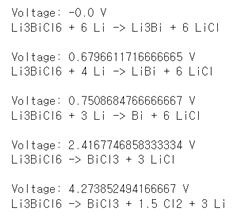# Error with get_element_profile

Hi, I would like to estimate the grand lithium potential for LiPS5Cl. Here is the code:

``````from pymatgen import MPRester, Composition, Element
from pymatgen.io.vasp import Vasprun
from pymatgen.analysis.phase_diagram import PhaseDiagram, CompoundPhaseDiagram, PDPlotter
from pymatgen.entries.computed_entries import ComputedEntry
from pymatgen.entries.compatibility import MaterialsProjectCompatibility
import json, re, palettable
import matplotlib as mpl
vasprun = Vasprun("vasprun.xml.relax2.gz")
entry = vasprun.get_computed_entry(inc_structure=True)

rester = MPRester(api_key='XXXXXXXXXXXXXXX')
mp_entries = rester.get_entries_in_chemsys(["Li", "P", "S", "Cl"])

#print(mp_entries)

with open("lpo_entries.json") as f:
lpo_entries = [ComputedEntry.from_dict(d) for d in lpo_data]

compatibility = MaterialsProjectCompatibility()
entry = compatibility.process_entry(entry)
entries = compatibility.process_entries([entry] + mp_entries + lpo_entries)
Li_entries = [e for e in entries  if e.composition.reduced_formula == "Li"]
uli0 = min(Li_entries, key=lambda e:e.energy_per_atom).energy_per_atom

el_profile = PhaseDiagram.get_element_profile(element=Element("Li"), comp=entry.composition)
for i, d in enumerate(el_profile):
voltage = -d(["chempot"] - uli0)
print("Voltage: %s V" % voltage)
print(d["reaction"])
print("")
``````

I got the following error:

``````el_profile = PhaseDiagram.get_element_profile(element=Element("Li"), comp=entry.composition)

TypeError: get_element_profile() missing 1 required positional argument: 'self'
``````

May you please tell me how to correct this code?

Hi @thienbinh92,

this is a classic error that I stumbled over many time, that has really nothing to do with pymatgen, but with python classes and their objects.
`get_element_profile` is not a static method of `PhaseDiagram`, so you can not call it without initializing a `PhaseDiagram` object first.

Here is a more minimal example (not requiring to load any data from files) of your code that works, but is probably useless, since I have not worked with pymatgen phase diagrams before and have no idea what I am actually doing:

``````from pymatgen.ext.matproj import MPRester
from pymatgen.core import Composition, Element
from pymatgen.analysis.phase_diagram import PhaseDiagram, PDEntry

rester = MPRester()
mp_entries = rester.get_entries_in_chemsys(["Li", "P", "S", "Cl"])
pd_entries = []
# here we create a list of PDEntries that are necessary to initialize
# the PhaseDiagram object
for entry in mp_entries:
pd_entries.append(PDEntry(composition=entry.composition,
energy=entry.energy))

# here we initialize the object wich needs a list of PDEntries
PD = PhaseDiagram(pd_entries)

comp = Composition("Li12 P4 S16")
#now we can call the get_element_profile method
el_profile = PD.get_element_profile(element=Element("Li"), comp=comp)
for i, d in enumerate(el_profile):
print(d["reaction"])
print("")
``````

P.S.: This assumes your MAPI key is set in ~/.pmgrc.yaml

1 Like

I use a few changes to find the reaction potential value, as follows:and then I change the composition by Li3ScCl6,Li3LuCl6 and get the same oxidation potential value (4.2738V) and I don’t know why there is this confusion. How can I fix this error? Thanks for your help!